Email us to get an instant 20% discount on highly effective K-12 Math & English kwizNET Programs!

#### Online Quiz (WorksheetABCD)

Questions Per Quiz = 2 4 6 8 10

### MEAP Preparation - Grade 4 Mathematics1.41 Types of Fractions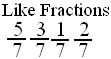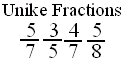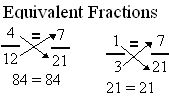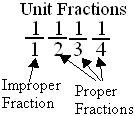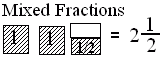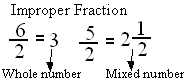Directions: Answer the following questions. Also write at least 5 examples of each type of fractions.
 Q 1: An improper fraction is sometimes a mixed number or sometimes a whole number.TrueFalse Q 2: Fractions with same denominators are calledunlike fractionslike fractions Q 3: A fraction less than 1 is called a proper fraction. A fraction equal to one and more than 1 is called _________.mixed fractionproper fractionimproper fraction Q 4: A number with a whole number and a proper fraction is aproper numbermixed number Q 5: Fraction with numerator 1 is called a unit fraction. Are all unit fractions proper?YesNo Q 6: A mixed number denotes the sum of a whole number-part and proper fraction part.FalseTrue Question 7: This question is available to subscribers only! Question 8: This question is available to subscribers only!